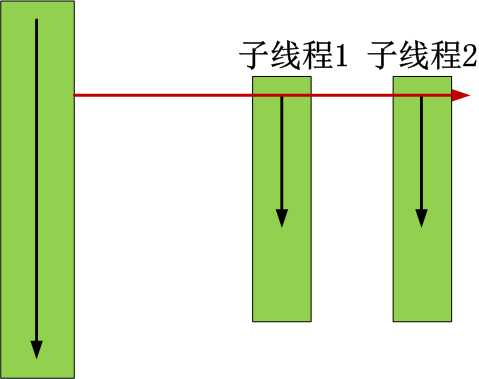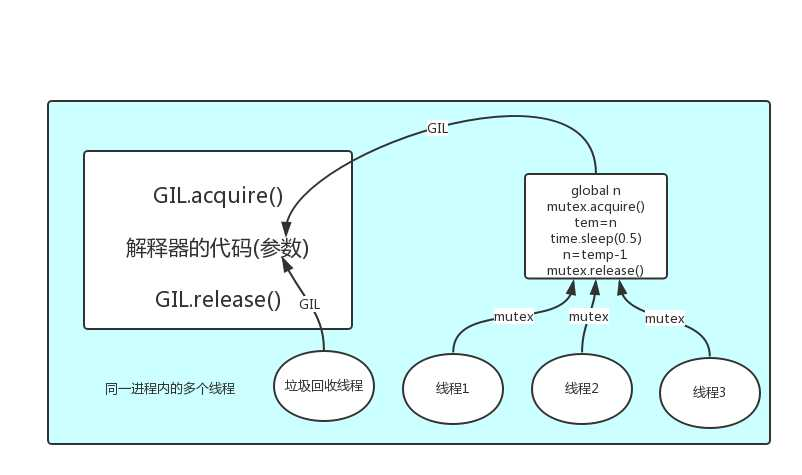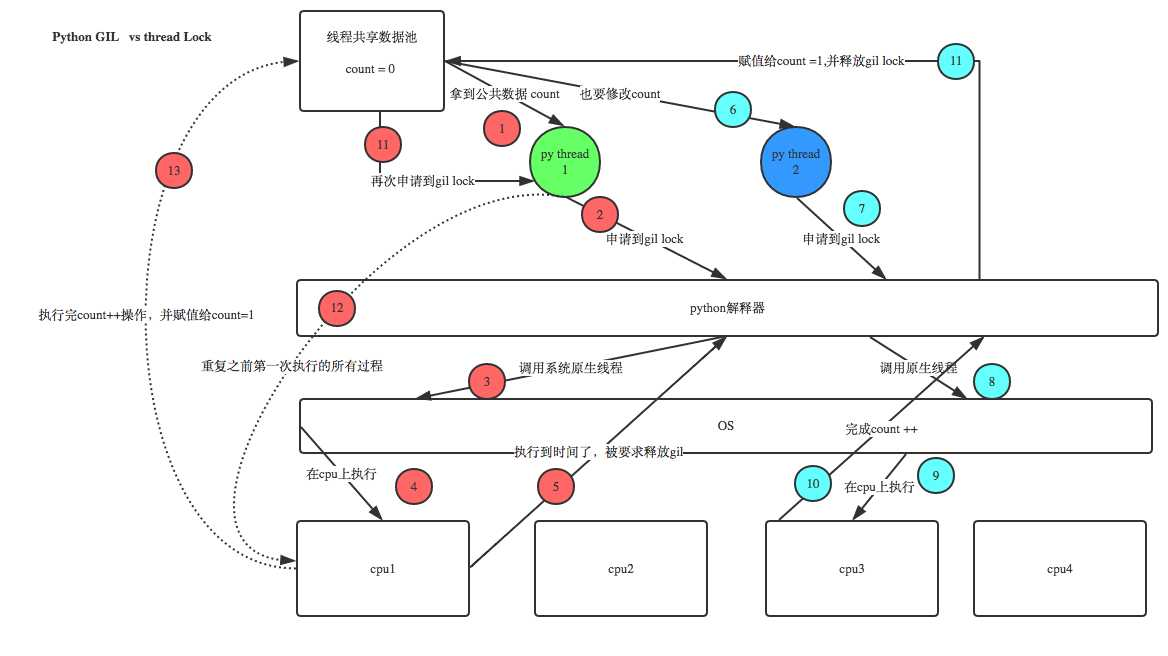# python开发线程:线程&守护线程&全局解释器锁

## 二 开启线程的两种方式```#方式一
import time
def sayhi(name):
time.sleep(2)
print(‘%s say hello‘ %name)

if __name__ == ‘__main__‘:
t.start()
print(‘主线程‘)``````#方式二
import time
def __init__(self,name):
super().__init__()
self.name=name
def run(self):
time.sleep(2)
print(‘%s say hello‘ % self.name)

if __name__ == ‘__main__‘:
t = Sayhi(‘egon‘)
t.start()
print(‘主线程‘)```## 三 在一个进程下开启多个线程与在一个进程下开启多个子进程的区别```from threading import Thread
from multiprocessing import Process
import os

def work():
print(‘hello‘)

if __name__ == ‘__main__‘:
#在主进程下开启线程
t.start()
print(‘主线程/主进程‘)
‘‘‘
打印结果:
hello
主线程/主进程
‘‘‘

#在主进程下开启子进程
t=Process(target=work)
t.start()
print(‘主线程/主进程‘)
‘‘‘
打印结果:
主线程/主进程
hello
‘‘‘```

1 谁的开启速度快```from threading import Thread
from multiprocessing import Process
import os

def work():
print(‘hello‘,os.getpid())

if __name__ == ‘__main__‘:
#part1:在主进程下开启多个线程,每个线程都跟主进程的pid一样
t1.start()
t2.start()
print(‘主线程/主进程pid‘,os.getpid())

#part2:开多个进程,每个进程都有不同的pid
p1=Process(target=work)
p2=Process(target=work)
p1.start()
p2.start()
print(‘主线程/主进程pid‘,os.getpid())```

2 瞅一瞅pid```from  threading import Thread
from multiprocessing import Process
import os
def work():
global n
n=0

if __name__ == ‘__main__‘:
# n=100
# p=Process(target=work)
# p.start()
# p.join()
# print(‘主‘,n) #毫无疑问子进程p已经将自己的全局的n改成了0,但改的仅仅是它自己的,查看父进程的n仍然为100

n=1
t.start()
t.join()
print(‘主‘,n) #查看结果为0,因为同一进程内的线程之间共享进程内的数据```

3 同一进程内的线程共享该进程的数据？

## 四 练习```#_*_coding:utf-8_*_
#!/usr/bin/env python
import multiprocessing

import socket
s=socket.socket(socket.AF_INET,socket.SOCK_STREAM)
s.bind((‘127.0.0.1‘,8080))
s.listen(5)

def action(conn):
while True:
data=conn.recv(1024)
print(data)
conn.send(data.upper())

if __name__ == ‘__main__‘:

while True:

p.start()``````#_*_coding:utf-8_*_
#!/usr/bin/env python

import socket

s=socket.socket(socket.AF_INET,socket.SOCK_STREAM)
s.connect((‘127.0.0.1‘,8080))

while True:
msg=input(‘>>: ‘).strip()
if not msg:continue

s.send(msg.encode(‘utf-8‘))
data=s.recv(1024)
print(data)``````from threading import Thread
msg_l=[]
format_l=[]
def talk():
while True:
msg=input(‘>>: ‘).strip()
if not msg:continue
msg_l.append(msg)

def format_msg():
while True:
if msg_l:
res=msg_l.pop()
format_l.append(res.upper())

def save():
while True:
if format_l:
with open(‘db.txt‘,‘a‘,encoding=‘utf-8‘) as f:
res=format_l.pop()
f.write(‘%s\n‘ %res)

if __name__ == ‘__main__‘:
t1.start()
t2.start()
t3.start()```

View Code

## 五 线程相关的其他方法

```Thread实例对象的方法
# isAlive(): 返回线程是否活动的。
# getName(): 返回线程名。
# setName(): 设置线程名。```from threading import Thread
from multiprocessing import Process
import os

def work():
import time
time.sleep(3)

if __name__ == ‘__main__‘:
#在主进程下开启线程
t.start()

print(‘主线程/主进程‘)

‘‘‘
打印结果:
主线程/主进程
‘‘‘```

View Code

```from threading import Thread
import time
def sayhi(name):
time.sleep(2)
print(‘%s say hello‘ %name)

if __name__ == ‘__main__‘:
t.start()
t.join()
print(‘主线程‘)
print(t.is_alive())
‘‘‘
egon say hello
主线程
False
‘‘‘```

## 六 守护线程

```#1.对主进程来说，运行完毕指的是主进程代码运行完毕

#2.对主线程来说，运行完毕指的是主线程所在的进程内所有非守护线程统统运行完毕，主线程才算运行完毕```

```#1 主进程在其代码结束后就已经算运行完毕了（守护进程在此时就被回收）,然后主进程会一直等非守护的子进程都运行完毕后回收子进程的资源(否则会产生僵尸进程)，才会结束，

#2 主线程在其他非守护线程运行完毕后才算运行完毕（守护线程在此时就被回收）。因为主线程的结束意味着进程的结束，进程整体的资源都将被回收，而进程必须保证非守护线程都运行完毕后才能结束。```
```from threading import Thread
import time
def sayhi(name):
time.sleep(2)
print(‘%s say hello‘ %name)

if __name__ == ‘__main__‘:
t.setDaemon(True) #必须在t.start()之前设置
t.start()

print(‘主线程‘)
print(t.is_alive())
‘‘‘
主线程
True
‘‘‘``````from threading import Thread
import time
def foo():
print(123)
time.sleep(1)
print("end123")

def bar():
print(456)
time.sleep(3)
print("end456")

t1.daemon=True
t1.start()
t2.start()
print("main-------")```

## 七 全局解释器锁GIL介绍

```‘‘‘

In CPython, the global interpreter lock, or GIL, is a mutex that prevents multiple
native threads from executing Python bytecodes at once. This lock is necessary mainly
because CPython’s memory management is not thread-safe. (However, since the GIL
exists, other features have grown to depend on the guarantees that it enforces.)
‘‘‘

### 7.1 GIL介绍

GIL本质就是一把互斥锁，既然是互斥锁，所有互斥锁的本质都一样，都是将并发运行变成串行，以此来控制同一时间内共享数据只能被一个任务所修改，进而保证数据安全。```‘‘‘
#验证python test.py只会产生一个进程
#test.py内容
import os,time
print(os.getpid())
time.sleep(1000)
‘‘‘
python3 test.py
#在windows下
#在linux下
ps aux |grep python```

```#1 所有数据都是共享的，这其中，代码作为一种数据也是被所有线程共享的（test.py的所有代码以及Cpython解释器的所有代码）

#2 所有线程的任务，都需要将任务的代码当做参数传给解释器的代码去执行，即所有的线程要想运行自己的任务，首先需要解决的是能够访问到解释器的代码。```### 7.2 GIL与Lock

GIL保护的是解释器级的数据，保护用户自己的数据则需要自己加锁处理，如下图### 7.3 GIL与多线程

```#1. cpu到底是用来做计算的，还是用来做I/O的？

#2. 多cpu，意味着可以有多个核并行完成计算，所以多核提升的是计算性能

#3. 每个cpu一旦遇到I/O阻塞，仍然需要等待，所以多核对I/O操作没什么用处```

```#分析：

#单核情况下，分析结果:
如果四个任务是计算密集型，没有多核来并行计算，方案一徒增了创建进程的开销，方案二胜
如果四个任务是I/O密集型，方案一创建进程的开销大，且进程的切换速度远不如线程，方案二胜

#多核情况下，分析结果：
如果四个任务是计算密集型，多核意味着并行计算，在python中一个进程中同一时刻只有一个线程执行用不上多核，方案一胜
如果四个任务是I/O密集型，再多的核也解决不了I/O问题，方案二胜

#结论：现在的计算机基本上都是多核，python对于计算密集型的任务开多线程的效率并不能带来多大性能上的提升，甚至不如串行(没有大量切换)，但是，对于IO密集型的任务效率还是有显著提升的。```

Begin typing your search term above and press enter to search. Press ESC to cancel.